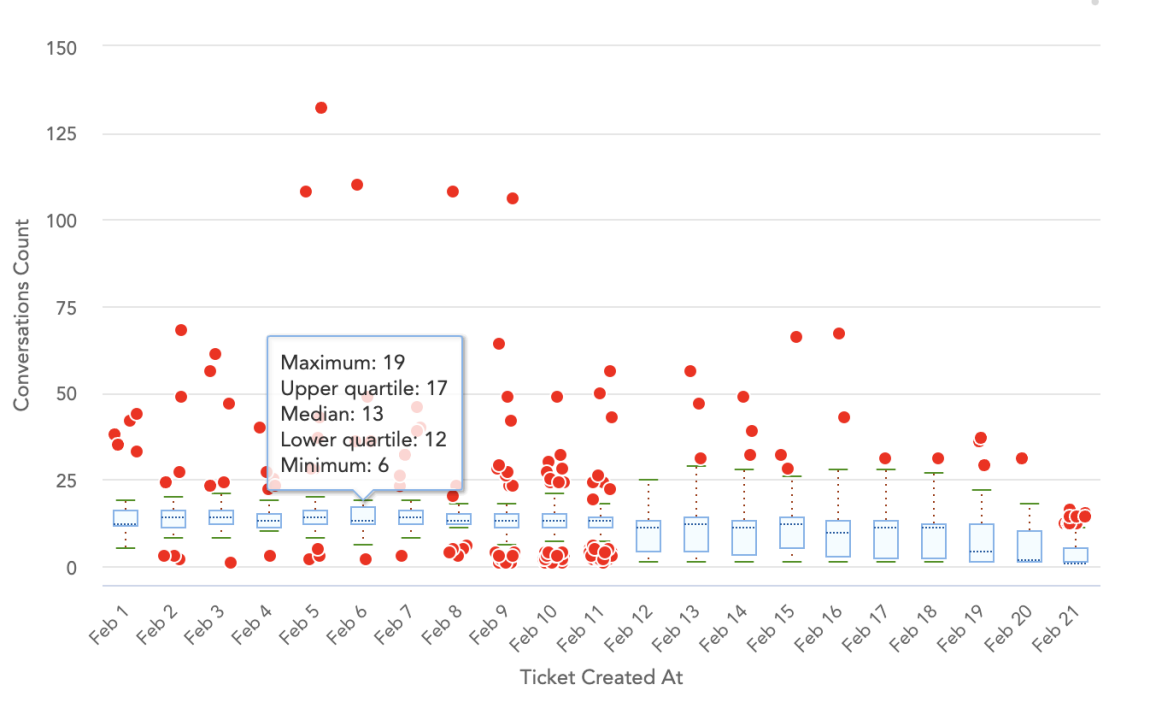# What is the purpose and use of chart view in patterns and outliers ?

Modified on Tue, 21 Mar 2023 at 09:30 AM

# Summary

Chart views are used to display the overall values of patterns and outliers. Below are definitions to help you interpret the results displayed in the Patterns and Outliers charts.

## Maximum, Minimum, Median, Upper Quartile, Lower Quartile :

• Maximum and minimum values are calculated according to the number of cases generated on the particular date(i.e., overall high case count will be calculated as maximum value and minimum case count will be denoted as minimum case count ).

• In this case, the median value will be calculated based on the average value of both the maximum and minimum case count values.

• With the help of the quartile formula, the upper quartile and lower quartile values are calculated. Same has been described on the below screenshot as a tile bar.

• Outlier cases are indicated by RED dots.